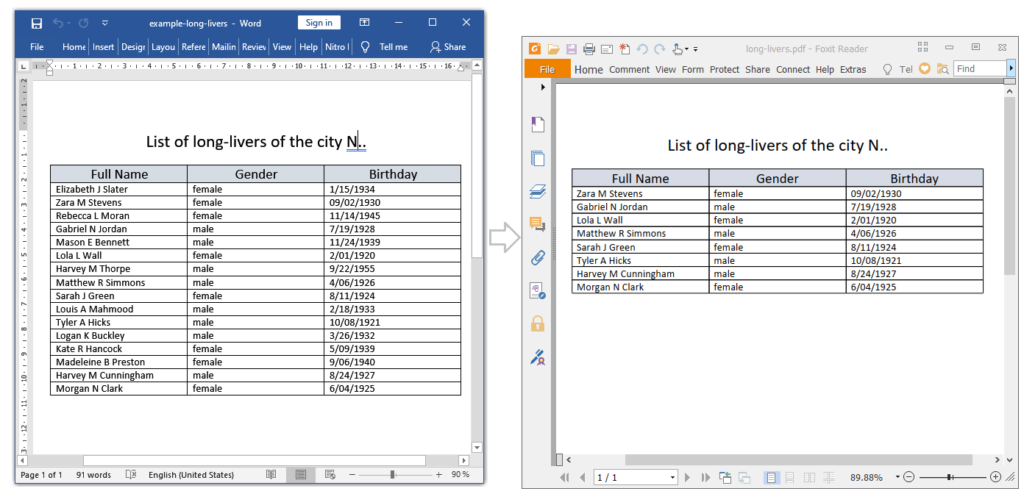Based on questions from our customers.

In this article, you’ll learn how else to work with tables. For example, we have a document with a table containing various persons with different age. The third cell in each row contains the person’s date of birth. You need to create a new document with a table that will contain a list of only elderly people aged 90 and older.

The following is required:

• Load a document with a table.
• Find a first table in the document.
• Loop by the all rows from the end.
• Take the 3rd cell with the birth date.
• Calculate the person age.
• Remove the row if it doesn’t contain a long-liver.
• Save the document as new PDF document.

The following code example shows how to do this.

```  public static void FindTextFromTable()
{
int longLiverMinYears = 90;

string inpFile = @"..\..\example.docx";
string outFile = Path.ChangeExtension(inpFile, ".pdf");

// Load a document with a table containing various persons with different age.

// Find a first table in the document.
Table table = (Table)dc.GetChildElements(true, ElementType.Table).First();

// Loop by the all rows from the end.
// Find long-livers.
bool isLongLiver = false;

for (int r = table.Rows.Count - 1; r > 0; r--)
{
isLongLiver = false;

// Take the 3rd cell with the birth date.
TableCell tc = table.Rows[r].Cells;

// Get the birth date.
DateTime birthDate = DateTime.Now;
if (DateTime.TryParse(tc.Content.ToString(), CultureInfo.CreateSpecificCulture("en-US"), DateTimeStyles.None, out birthDate))
{
// Get the person age.
// Remove the row if the person isn't long-liver.
if (CalculateAge(birthDate) >= longLiverMinYears)
isLongLiver = true;
}
// Remove the row if it doesn't contain a long-liver.
if (!isLongLiver)
table.Rows.RemoveAt(r);
}

// Save the document as PDF.
dc.Save(outFile, new PdfSaveOptions());

// Open the result for demonstration purposes.
System.Diagnostics.Process.Start(new System.Diagnostics.ProcessStartInfo(outFile) { UseShellExecute = true });
}
private static int CalculateAge(DateTime dateOfBirth)
{
int age = 0;
age = DateTime.Now.Year - dateOfBirth.Year;
if (DateTime.Now.DayOfYear < dateOfBirth.DayOfYear)
age = age - 1;
return age;
}```

As a result:The complete code example see: https://www.sautinsoft.com/products/document/examples/from-customers-find-text-from-table-net-csharp-vb.php